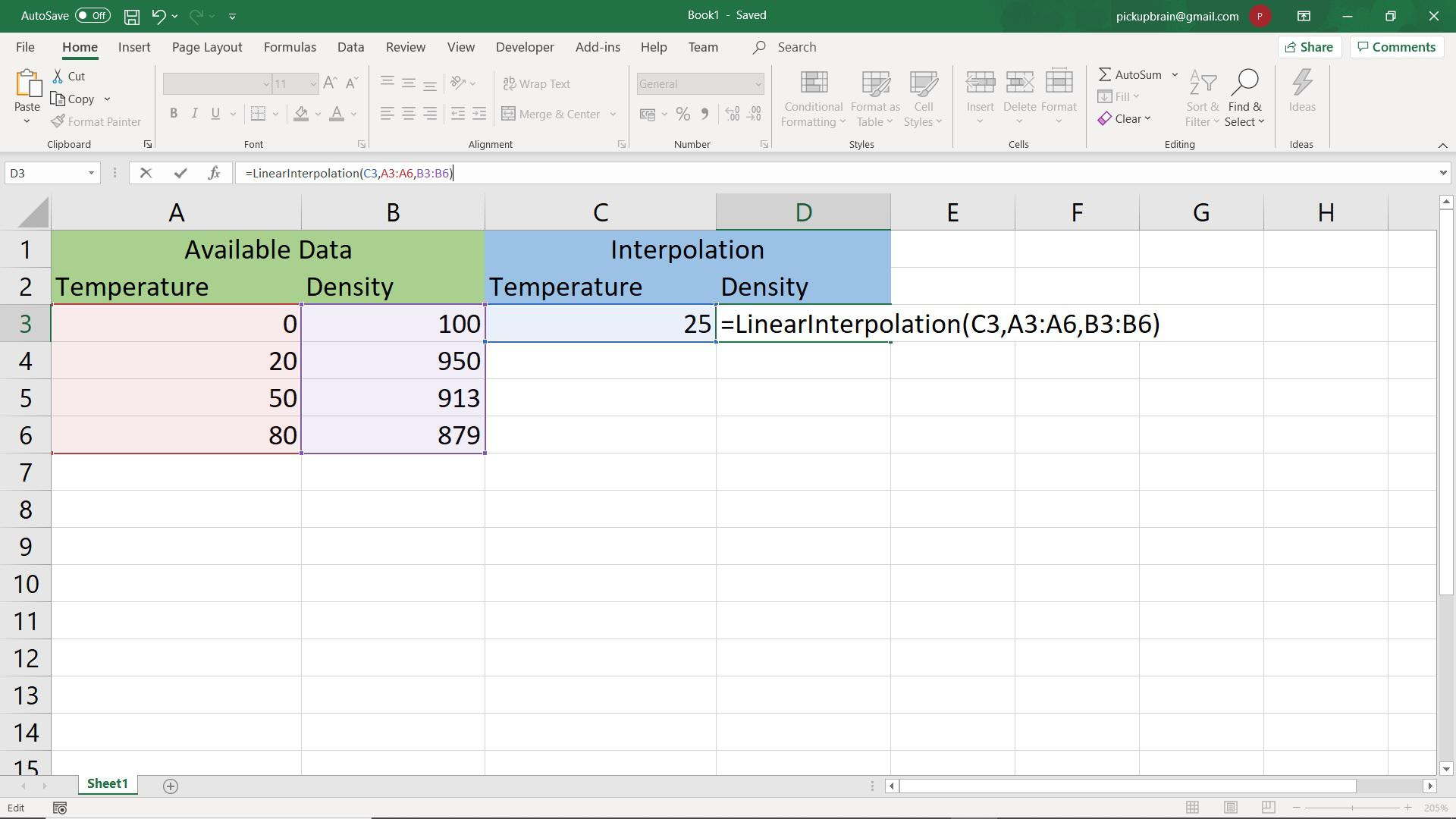# Automated interpolation formula for Excel: Define excel interpolate function & use it foreverInterpolation is a way to estimate values between two points of a data set. Most of the real-world data are available as discrete points. Interpolation comes handy if you want to estimate the data between two points. The most simple interpolation is a linear interpolation, where variation of data between two data points is assumed linear.

Let us consider the following example. We have of density of water at different temperature. Using this data, we need to find density at intermediate temperature, say 25°C or so. One simple method is to use density at the 20°C and 50 °C to estimate value at the intermediate temperature of 25°C using linear interpolation.

## Interpolation in Excel

### Linear Interpolation

Following interpolation steps describes how to estimate specific heat at 25°C from the above known data.

• Step 1: Finding nearest lower and upper known data, i.e. density at 20°C (x1) and 50°C (x2) is 950 (y1) and 913 (y2) kg/m3.
• Step 2: Interpolating value (y) at 25°C (x) using $y = \frac{y_2 – y_1}{x_2-x_1} \times x + y_1$

### Visual Basic function for Linear Interpolation in Excel

Once the nearest lower and upper known data, writing interpolation function is is easy.

Following code Visual Basis function (LinInterp) takes known x and y values in variable x_values and y_values along with x where we need to calculate y using interpolation.

Function LinearInterpolation(x, xvalues, yvalues)

x1 = Application.WorksheetFunction.Index(xvalues, Application.WorksheetFunction.Match(x, xvalues, 1))
x2 = Application.WorksheetFunction.Index(xvalues, Application.WorksheetFunction.Match(x, xvalues, 1) + 1)
y1 = Application.WorksheetFunction.Index(yvalues, Application.WorksheetFunction.Match(x, xvalues, 1))
y2 = Application.WorksheetFunction.Index(yvalues, Application.WorksheetFunction.Match(x, xvalues, 1) + 1)

LinearInterpolation = y1 + (y2 - y1) * (x - x1) / (x2 - x1)

End Function


## Writing user defined Interpolation function in Excel

To make the above LinearInterpolation function available in excel like other functions, you need to carry out the following steps:

### Step 1: Open Visual basic Editor

If developer tab is not available in your Excel, you need to go to Files > Options, click Customize Ribbon and check the box against Developer in Main Tabs. This will activate Developer tab in your Excel.

Now, Open Visual basic Editor by navigating to Developer tab > Visual Basic

### Step 2: Create Module

To create new Module, navigate Insert Tab and click Module. This opens up a new window where you can write Visual Basic Code.

### Step 3: Write LinearInterpolation Function

Now, copy the LinearInterpolation code written above into the newly opened Modules window and then close the module window and visual basic editor window.

Now, you can test LinearInterpolation(x, xvalues, yvalues) function in current Excel Workbook, where x is the value for which we need to estimate y using interpolation on data which has xvalues and yvalues. Currently, the above function is only available for the present workbook.

### Step 4: Save Excel as Addin to make it available to every one

Saving the current file as Addin is an important step to make it available to every Excel workbook. Save the current workbook as “Excel Addins” in the default folder which Windows prompts with the name “LinearInterpolation Function.xlam”. We will use this file name in the last step.

### Activating Excel Addin for Linear Interpolation

This is the last step of the process. It will make sure that LinearInterpolation function is available to every work book in the system. This is done by activating Excel Addin we made.

To activate add-in, navigate Files > Options > Add-in and select line ending with “LinearInterpolation Function.xlam” from the list of Inactive Add-in and click Go.

Enjoy!!!!